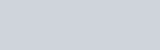# Best Maths for Machine Learning/AI/DL/DS Online Courses, Training with Certification-2022 Updated

Students who are searching for the Best Maths for Machine Learning/AI/DL/DS online courses then this is the right place to learn the course. Mathematics is having huge demand in artificial intelligence, machine learning, deep learning etc. From this  maths courses you will learn the concepts like Mathematical foundation, linear algebra, how to implement the algorithms in the python, probability theory, multivariate calculus, basics of the integrations, mathematics importance and benefits in machine learning and artificial intelligence, different algorithms of machine learning, special random variables, problems of machine learning and so on. Nowadays there are multiple number of Maths for Machine Learning/AI/DL/DS online courses in the world from them our experts of panel has picked out some Best Maths for Machine Learning/AI/DL/DS online courses for you which are listed below.

## #1 Mathematics for Machine Learning Specialization – Coursera

Mathematics for Machine Learning Specialization is offered by the Imperial college located in London. This specialisation is useful for learning the prerequisites of mathematics which are necessary for applications in machine learning and data science. More than 25k+ students are enrolled for this course. And you can see hands on project in every specialisation. After the completion of the specialisation you will get a course completion certificate. And in this specialisation there are 3 courses. In the 1st course you will learn about the linear algebra, in the 2nd course you will learn about the multivariate calculus, in the 3rd course you will learn about the Principal Component Analysis.
Key points:

• This specialisation contains three courses in each course you will learn deeply about the topic of mathematics for machine learning like linear algebra, multivariate calculus,  Principal Component Analysis.
• In the 1st course of this specialisation you will learn about what actually is the linear algebra and how it works and how it is related to the vectors and matrices and how o apply the various concepts of linear algebra to the machine learning.
• The 2nd course of this specialisation helps you in learning the multivariate calculus and how to calculate the vectors and how calculus is used to build the approximations to functions.
• In the 3rd course you will learn about the mathematical foundations which are used to derive the principal component analysis, and also you will learn some of the basic statistics of the data sets like mean values and variances etc.
• After completion of this Specialization you will be gained with the knowledge of prerequisite mathematical

Rating: 4.2 Out of 5
You can Signup here <=>

## #2 Mathematical Foundation For Machine Learning and AI – Udemy

People who want to learn about the mathematical tools which are used in the machine learning and AI, then you can take this Mathematical foundation for Artificial intelligence and machine learning online course which is created by Eduonix Learning Solutions academy. Here more than 3k candidates are learning this mathematics foundation course. Students will recall all the concepts of mathematics for the machine learning and AI. you people will come to know that how the implementation process of algorithms is done in the python. Instructor discussed how the topics are extended to the real world problems of machine learning. Here students will learn about the linear algebra, probability theory and multivariate calculus.
Key points:

• From the linear algebra section students will learn topics such as tensors, scalars, matrices, vectors, matrices and vectors norms, tensors and matrices in python, eigenvectors and eigenvalues, eigen decomposition, special matrices etc.
• From the multivariate calculus section instructors will explain about the introduction to the derivatives, basics of the integrations, gradients, optimization of convex, differential operators and gradient visualizations.
• From the probability theory section you people will understand the concepts such as probability theory introduction, probability distributions, covariance, variance, exceptations, graphing the probability distribution in the R, special random variables, covariance matrices in R etc.
• Students are able to know the importance of mathematics in machine learning and artificial intelligence. You will understand the structure of different algorithms of machine learning (ML).
• You people will learn that how the neural networks are placed together and know how those networks are operated.

Rating: 3.9 Out of 5
You can Signup here <=>

Conclusion:
From this Maths for Machine Learning/AI/DL/DS online courses students will gain more knowledge on mathematics. People who have to learn this maths online course then choose any course from the above mentioned courses. You will get the best job opportunities by learning this Maths for Machine Learning/AI/DL/DS online courses like machine learning engineer, data scientist, solution architect, front end developer, back end software development engineer, machine learning and artificial intelligence engineer and so on. Students can able to solve the problems in machine learning, AI, DL. here trainers will definitely clear out all your queries on maths. Please share this Maths for Machine Learning/AI/DL/DS article with your friends, colleagues through hike, linkedIn, whatsapp, instagram,twitter etc.
We Advice you to learn via Online Courses, Rather than Books, But We Suggest you use Books Only for reference purpose
8.6 Total Score
Best Maths for Machine Learning/AI/DL/DS Online Courses

Best Maths for Machine Learning/AI/DL/DS Online Courses

User Rating: No Ratings Yet!

We will be happy to hear your thoughts